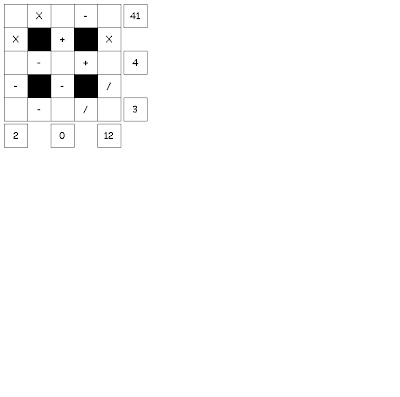## Friday 11 January 2013

### NUMBERS IN THE BIBLETry to fill in the missing numbers. Use the numbers 1 through 9 to complete the equations. Each number is only used once. Each row is a mathematics equation. Each column is a mathematics equation. Remember that multiplication and division are performed before addition and subtraction. THE MISSION DISCOURSE TO THE TWELVE IN THE GOSPEL OF LUKE IS IN THIS CHAPTER THIS IS THE NUMBER OF DISCOURSES IN MATTHEW LOOK AT MK 13:27 FOR THIS NUMBER SEE DEUTERONOMY 6:4 FOR THIS NUMBER THE NUMBER OF TIMES JESUS PRAYED IN GETHSEMANE IN MATTHEW SEE JN 19:14 AND ALSO MT 27:45 ACTS 6:3 WILL GIVE YOU THIS NUMBER SEE GENESIS 21:4 HOW MANY FISH IN THE FIRST FEEDING?